# Lakhmir Singh Solutions For Class 8 Science Chapter 16 Light

Light is a form of energy which makes things visible. We are able to see this beautiful world because of light. Light has an important role in our daily life because eyes cannot see any objects in the dark without a beam of light. So light enables us to see things from which it comes or from which it is reflected.

There are two types of lights, luminous and non-luminous light. The object which emits their own light is called luminous light like stars, sun and bulb. The objects that do not emit light are called non-luminous like a table, chair etc. many of the objects around us are seen by the reflected light.

This chapter explains how objects are visible by our naked eye, the importance of light, terms related to lights, the principle behind the vision of objects and how the human eyes are constructed.

## Download PDF Of Lakhmir Singh Science Class 8 Solutions For Chapter 16 Light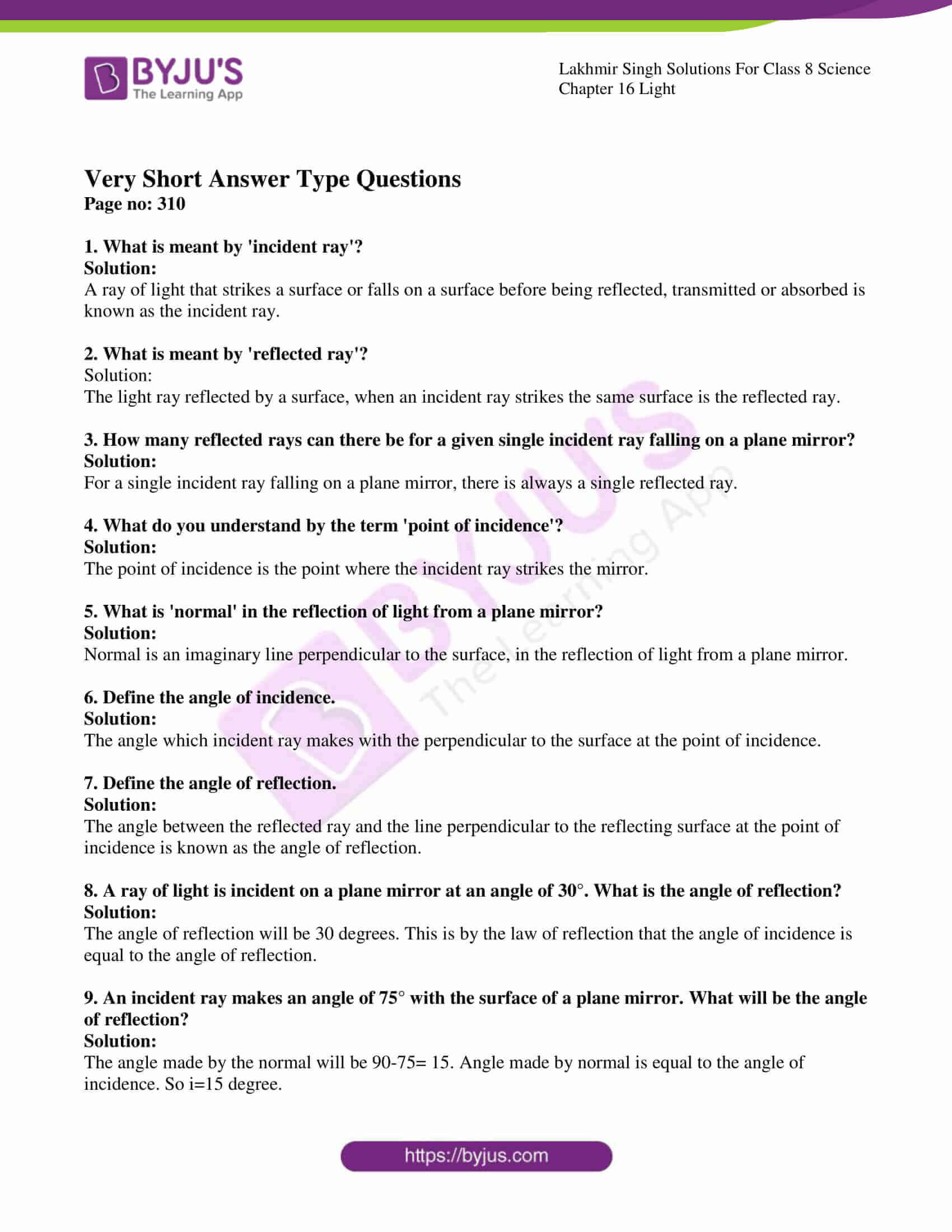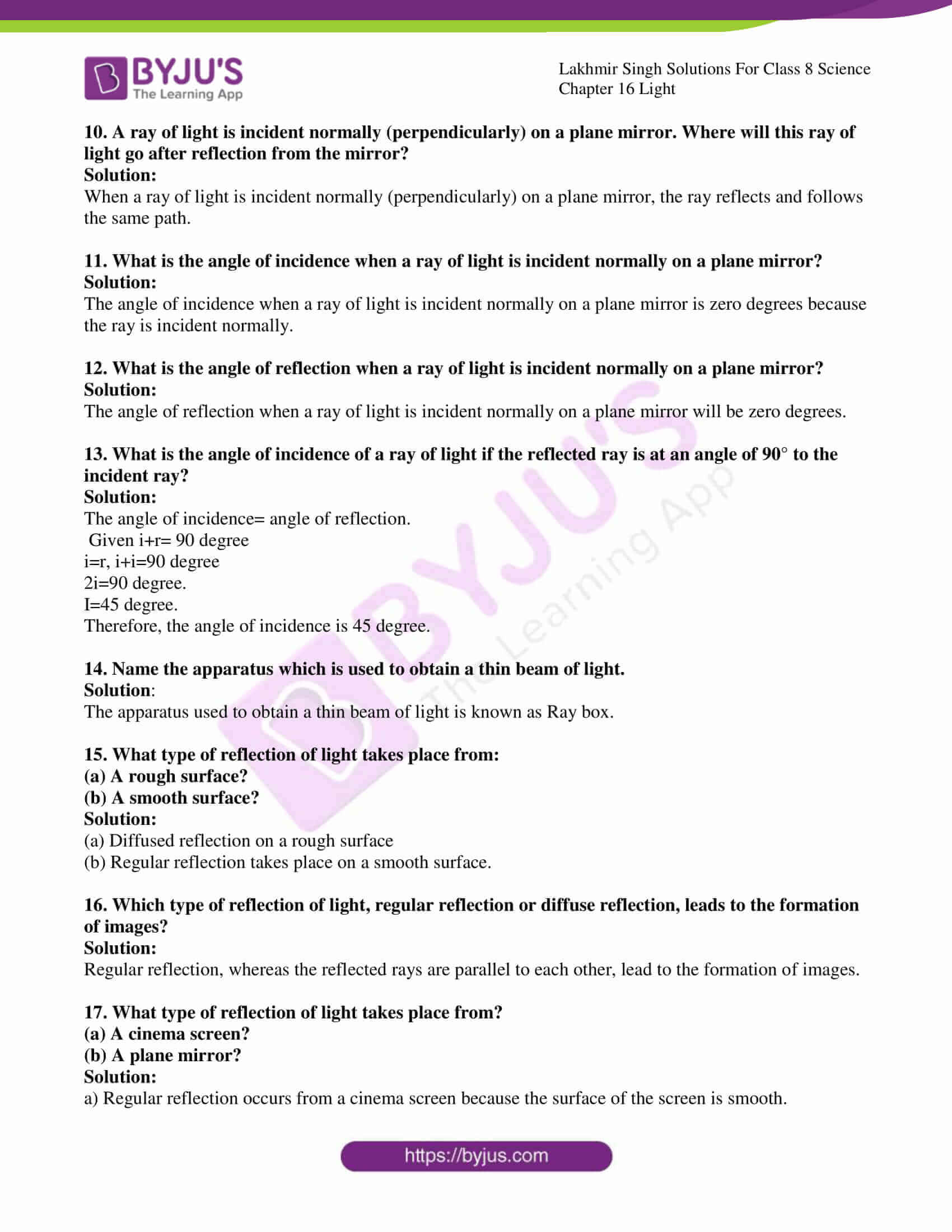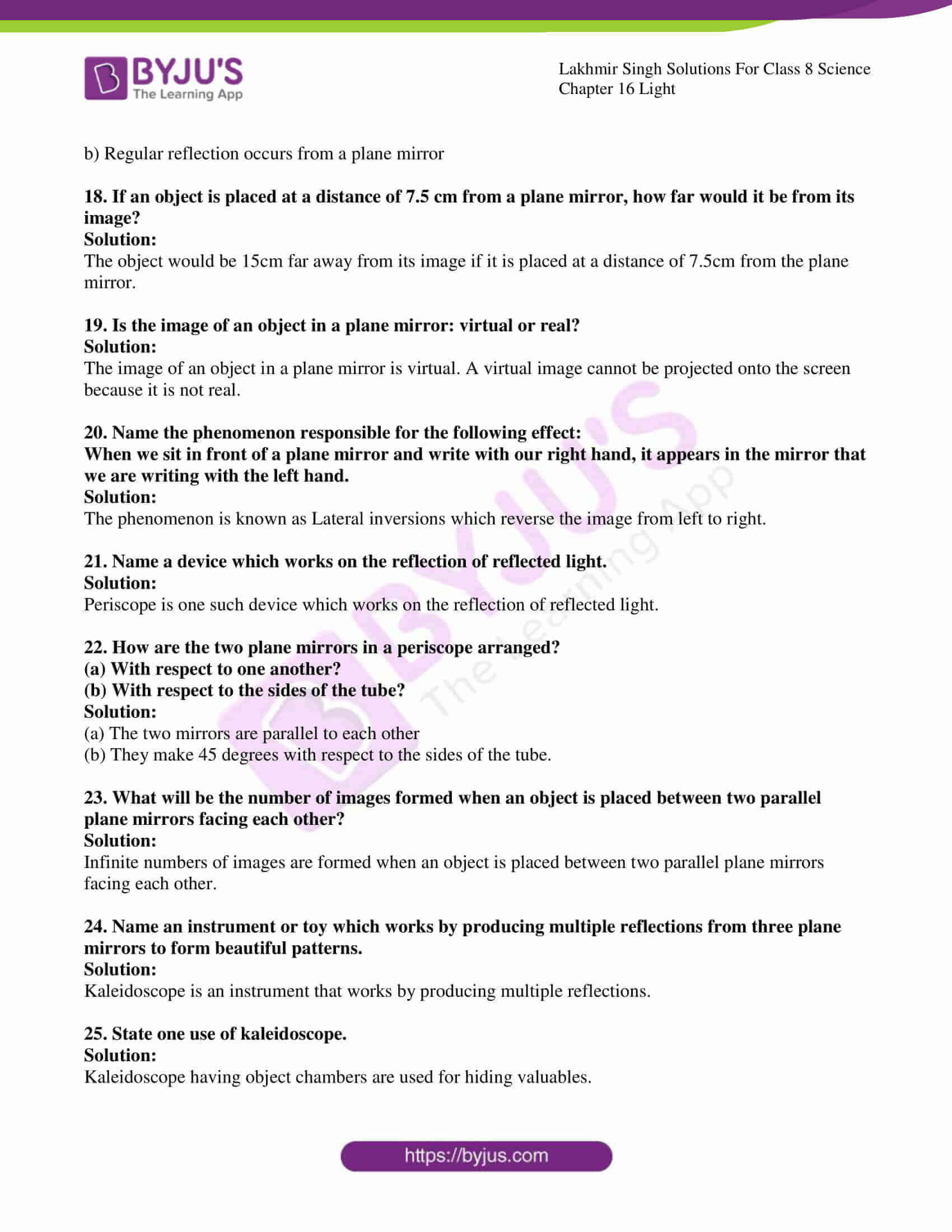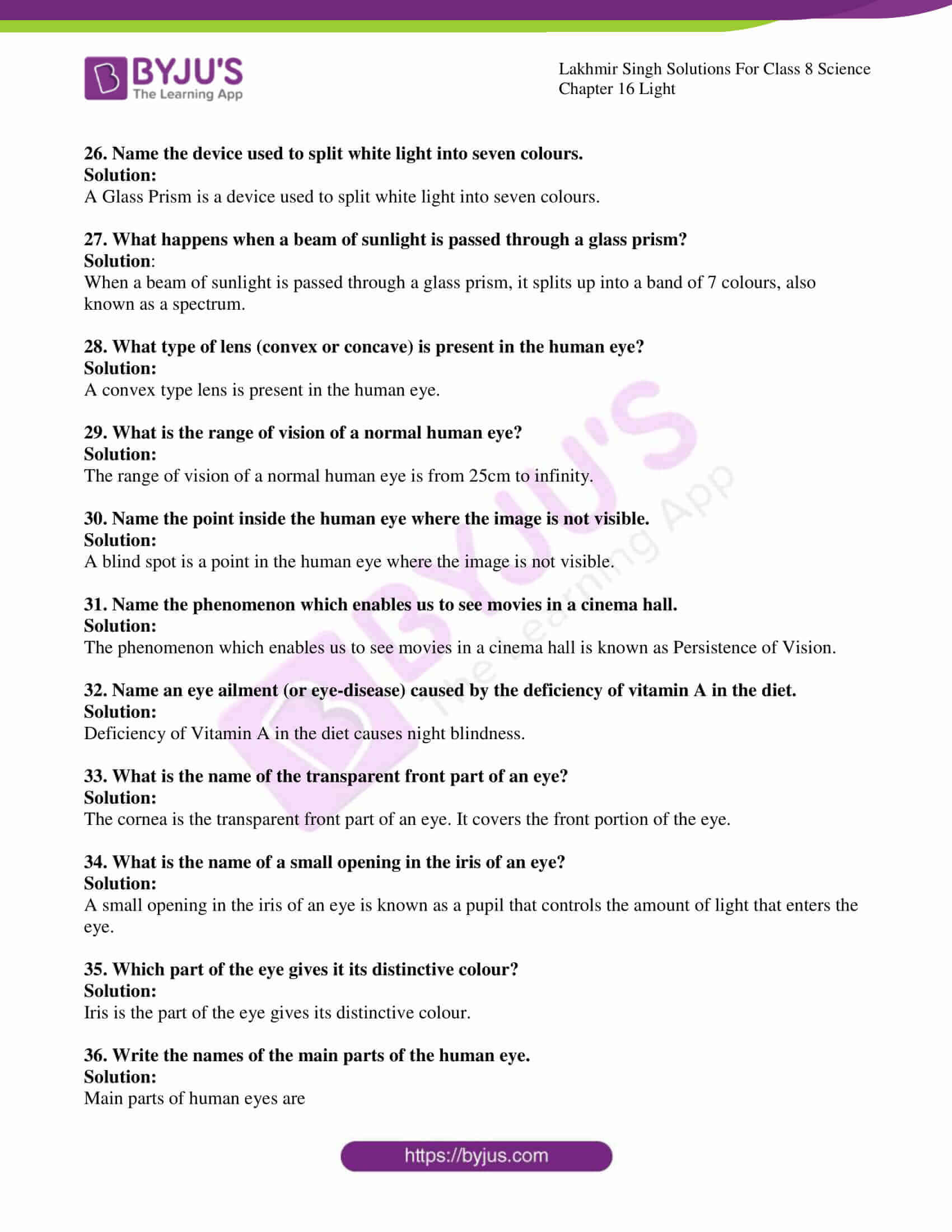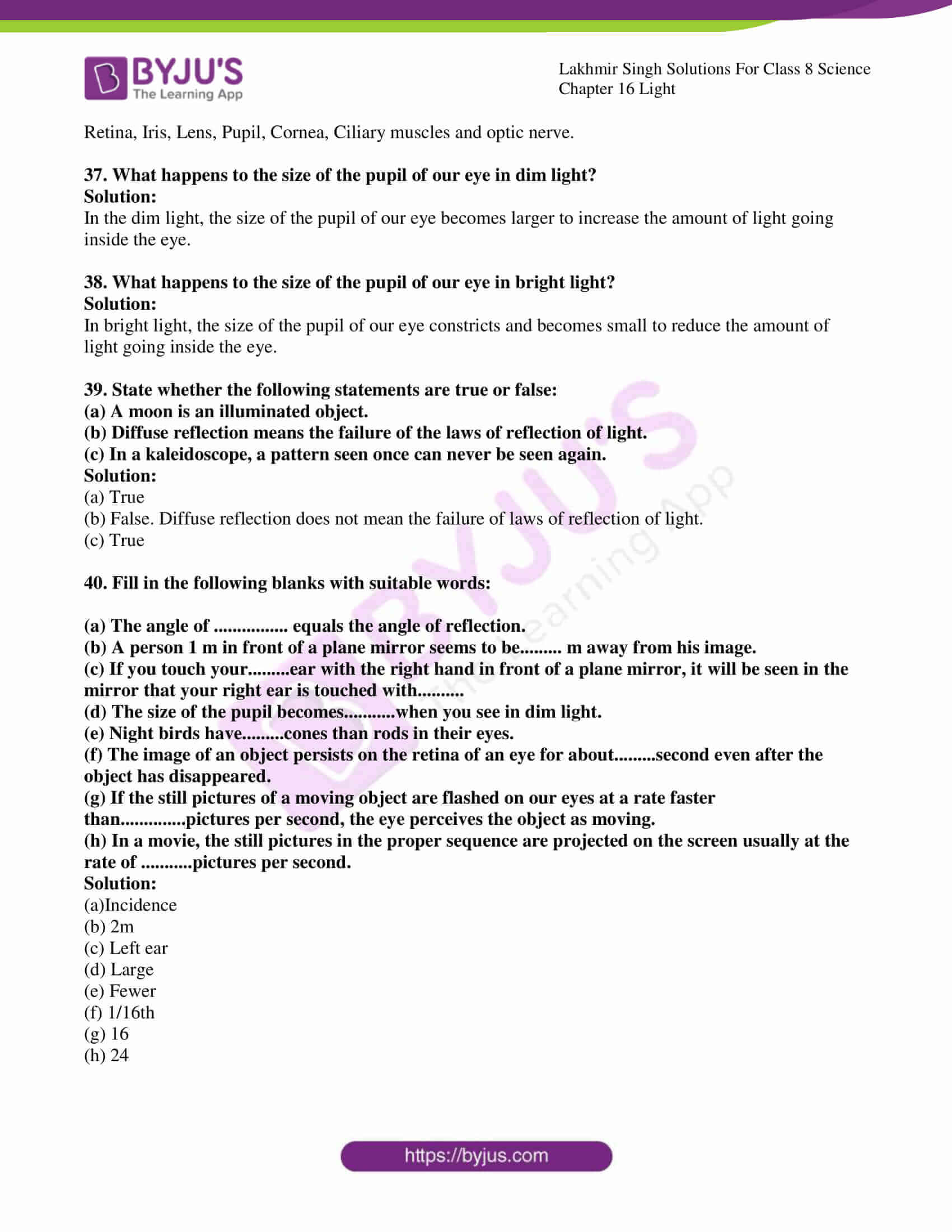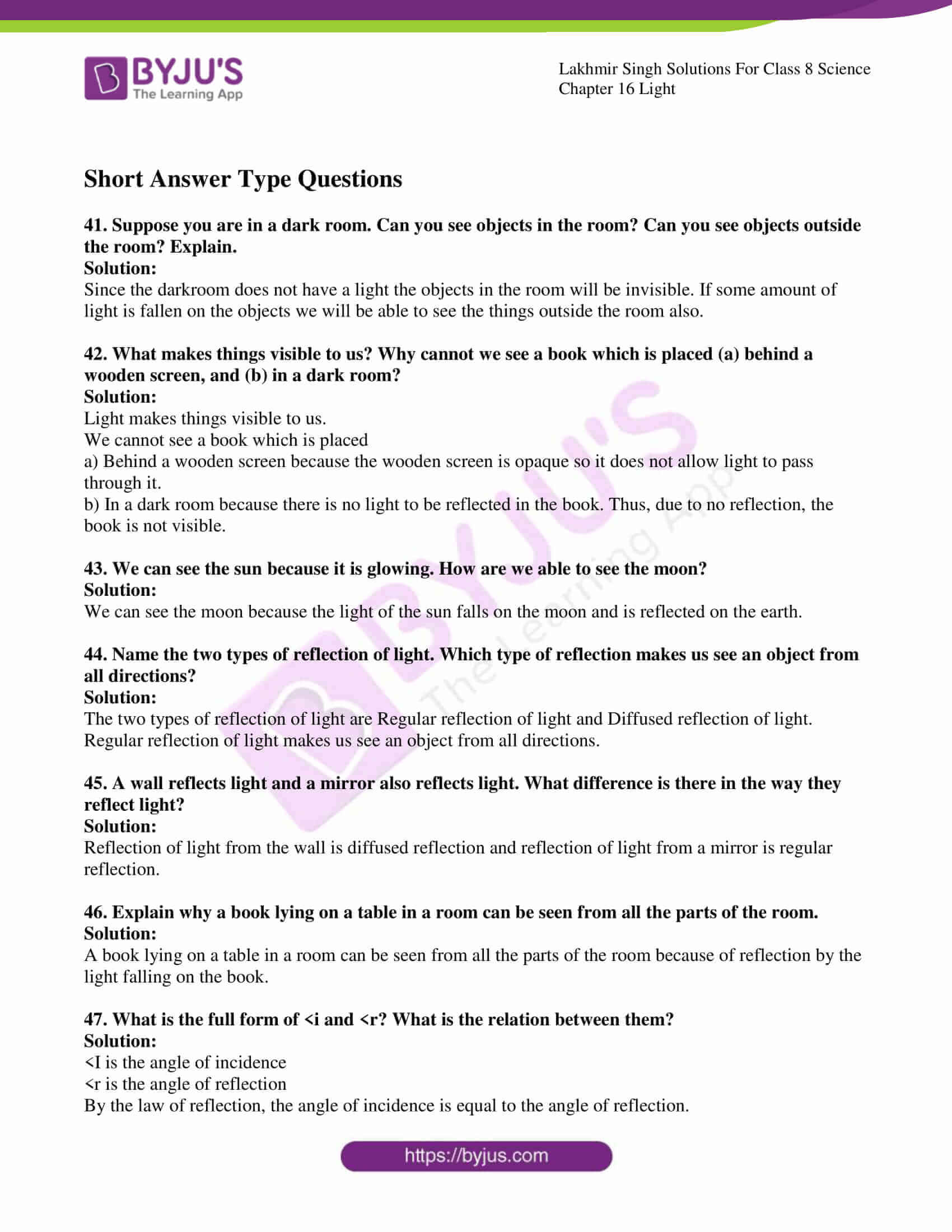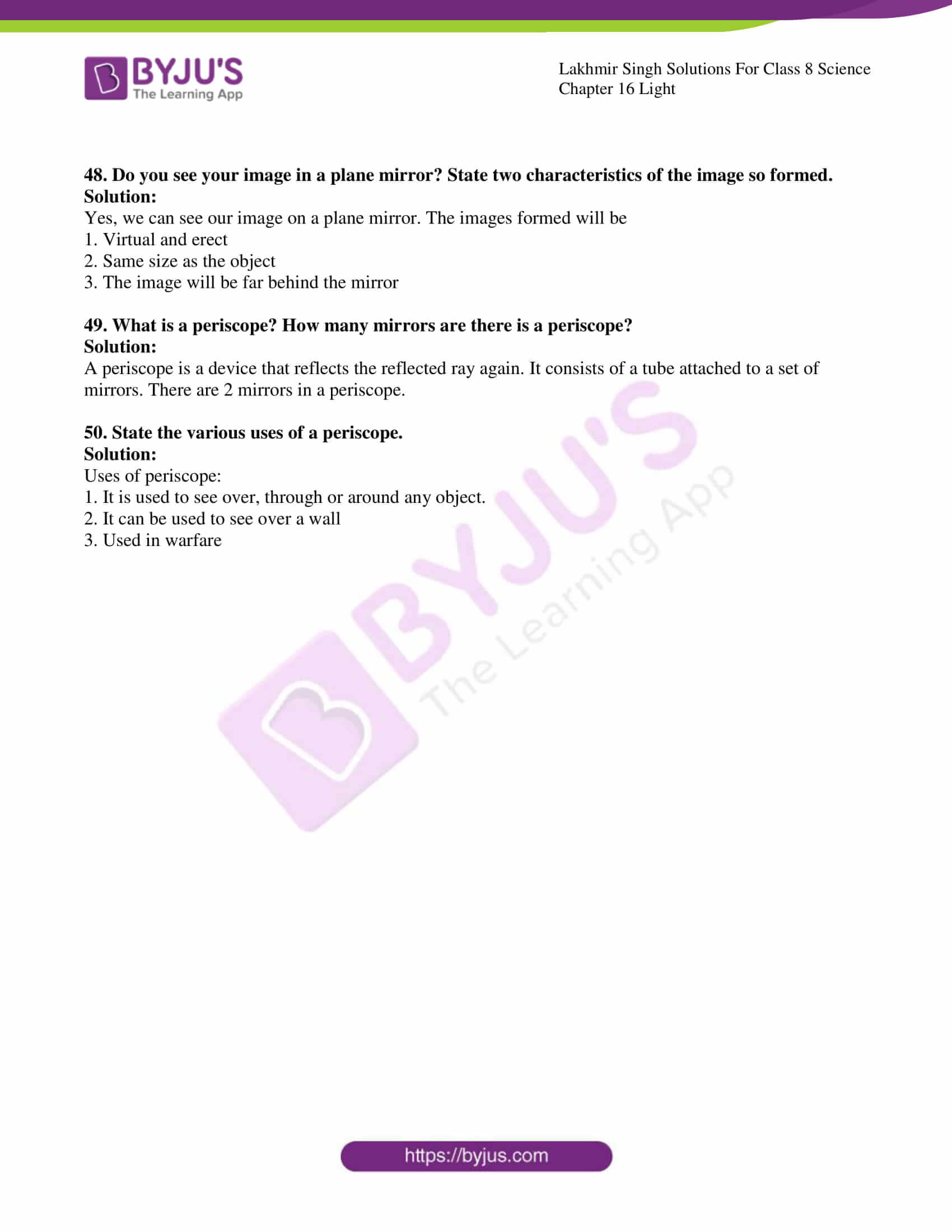### Access Lakhmir Singh Science Class 8 Solutions For Chapter 16 Light

Page no: 310

1. What is meant by ‘incident ray’?

Solution:

A ray of light that strikes a surface or falls on a surface before being reflected, transmitted or absorbed is known as the incident ray.

2. What is meant by ‘reflected ray’?

Solution:

The light ray reflected by a surface, when an incident ray strikes the same surface is the reflected ray.

3. How many reflected rays can there be for a given single incident ray falling on a plane mirror?

Solution:

For a single incident ray falling on a plane mirror, there is always a single reflected ray.

4. What do you understand by the term ‘point of incidence’?

Solution:

The point of incidence is the point where the incident ray strikes the mirror.

5. What is ‘normal’ in the reflection of light from a plane mirror?

Solution:

Normal is an imaginary line perpendicular to the surface, in the reflection of light from a plane mirror.

6. Define the angle of incidence.

Solution:

The angle which incident ray makes with the perpendicular to the surface at the point of incidence.

7. Define the angle of reflection.

Solution:

The angle between the reflected ray and the line perpendicular to the reflecting surface at the point of incidence is known as the angle of reflection.

8. A ray of light is incident on a plane mirror at an angle of 30°. What is the angle of reflection?

Solution:

The angle of reflection will be 30 degrees. This is by the law of reflection that the angle of incidence is equal to the angle of reflection.

9. An incident ray makes an angle of 75° with the surface of a plane mirror. What will be the angle of reflection?

Solution:

The angle made by the normal will be 90-75= 15. Angle made by normal is equal to the angle of incidence. So i=15 degree.

10. A ray of light is incident normally (perpendicularly) on a plane mirror. Where will this ray of light go after reflection from the mirror?

Solution:

When a ray of light is incident normally (perpendicularly) on a plane mirror, the ray reflects and follows the same path.

11. What is the angle of incidence when a ray of light is incident normally on a plane mirror?

Solution:

The angle of incidence when a ray of light is incident normally on a plane mirror is zero degrees because the ray is incident normally.

12. What is the angle of reflection when a ray of light is incident normally on a plane mirror?

Solution:

The angle of reflection when a ray of light is incident normally on a plane mirror will be zero degrees.

13. What is the angle of incidence of a ray of light if the reflected ray is at an angle of 90° to the incident ray?

Solution:

The angle of incidence= angle of reflection.

Given i+r= 90 degree

i=r, i+i=90 degree

2i=90 degree.

I=45 degree.

Therefore, the angle of incidence is 45 degree.

14. Name the apparatus which is used to obtain a thin beam of light.

Solution:

The apparatus used to obtain a thin beam of light is known as Ray box.

15. What type of reflection of light takes place from:

(a) A rough surface?

(b) A smooth surface?

Solution:

(a) Diffused reflection on a rough surface

(b) Regular reflection takes place on a smooth surface.

16. Which type of reflection of light, regular reflection or diffuse reflection, leads to the formation of images?

Solution:

Regular reflection, whereas the reflected rays are parallel to each other, lead to the formation of images.

17. What type of reflection of light takes place from?

(a) A cinema screen?

(b) A plane mirror?

Solution:

a) Regular reflection occurs from a cinema screen because the surface of the screen is smooth.

b) Regular reflection occurs from a plane mirror

18. If an object is placed at a distance of 7.5 cm from a plane mirror, how far would it be from its image?

Solution:

The object would be 15cm far away from its image if it is placed at a distance of 7.5cm from the plane mirror.

19. Is the image of an object in a plane mirror: virtual or real?

Solution:

The image of an object in a plane mirror is virtual. A virtual image cannot be projected onto the screen because it is not real.

20. Name the phenomenon responsible for the following effect:

When we sit in front of a plane mirror and write with our right hand, it appears in the mirror that we are writing with the left hand.

Solution:

The phenomenon is known as Lateral inversions which reverse the image from left to right.

21. Name a device which works on the reflection of reflected light.

Solution:

Periscope is one such device which works on the reflection of reflected light.

22. How are the two plane mirrors in a periscope arranged?

(a) With respect to one another?

(b) With respect to the sides of the tube?

Solution:

(a) The two mirrors are parallel to each other

(b) They make 45 degrees with respect to the sides of the tube.

23. What will be the number of images formed when an object is placed between two parallel plane mirrors facing each other?

Solution:

Infinite numbers of images are formed when an object is placed between two parallel plane mirrors facing each other.

24. Name an instrument or toy which works by producing multiple reflections from three plane mirrors to form beautiful patterns.

Solution:

Kaleidoscope is an instrument that works by producing multiple reflections.

25. State one use of kaleidoscope.

Solution:

Kaleidoscope having object chambers are used for hiding valuables.

26. Name the device used to split white light into seven colours.

Solution:

A Glass Prism is a device used to split white light into seven colours.

27. What happens when a beam of sunlight is passed through a glass prism?

Solution:

When a beam of sunlight is passed through a glass prism, it splits up into a band of 7 colours, also known as a spectrum.

28. What type of lens (convex or concave) is present in the human eye?

Solution:

A convex type lens is present in the human eye.

29. What is the range of vision of a normal human eye?

Solution:

The range of vision of a normal human eye is from 25cm to infinity.

30. Name the point inside the human eye where the image is not visible.

Solution:

A blind spot is a point in the human eye where the image is not visible.

31. Name the phenomenon which enables us to see movies in a cinema hall.

Solution:

The phenomenon which enables us to see movies in a cinema hall is known as Persistence of Vision.

32. Name an eye ailment (or eye-disease) caused by the deficiency of vitamin A in the diet.

Solution:

Deficiency of Vitamin A in the diet causes night blindness.

33. What is the name of the transparent front part of an eye?

Solution:

The cornea is the transparent front part of an eye. It covers the front portion of the eye.

34. What is the name of a small opening in the iris of an eye?

Solution:

A small opening in the iris of an eye is known as a pupil that controls the amount of light that enters the eye.

35. Which part of the eye gives it its distinctive colour?

Solution:

Iris is the part of the eye gives its distinctive colour.

36. Write the names of the main parts of the human eye.

Solution:

Main parts of human eyes are

Retina, Iris, Lens, Pupil, Cornea, Ciliary muscles and optic nerve.

37. What happens to the size of the pupil of our eye in dim light?

Solution:

In the dim light, the size of the pupil of our eye becomes larger to increase the amount of light going inside the eye.

38. What happens to the size of the pupil of our eye in bright light?

Solution:

In bright light, the size of the pupil of our eye constricts and becomes small to reduce the amount of light going inside the eye.

39. State whether the following statements are true or false:

(a) A moon is an illuminated object.

(b) Diffuse reflection means the failure of the laws of reflection of light.

(c) In a kaleidoscope, a pattern seen once can never be seen again.

Solution:

(a) True

(b) False. Diffuse reflection does not mean the failure of laws of reflection of light.

(c) True

40. Fill in the following blanks with suitable words:

(a) The angle of ……………. equals the angle of reflection.

(b) A person 1 m in front of a plane mirror seems to be……… m away from his image.

(c) If you touch your………ear with the right hand in front of a plane mirror, it will be seen in the mirror that your right ear is touched with……….

(d) The size of the pupil becomes………..when you see in dim light.

(e) Night birds have………cones than rods in their eyes.

(f) The image of an object persists on the retina of an eye for about………second even after the object has disappeared.

(g) If the still pictures of a moving object are flashed on our eyes at a rate faster than…………..pictures per second, the eye perceives the object as moving.

(h) In a movie, the still pictures in the proper sequence are projected on the screen usually at the rate of ………..pictures per second.

Solution:

(a)Incidence

(b) 2m

(c) Left ear

(d) Large

(e) Fewer

(f) 1/16th

(g) 16

(h) 24

41. Suppose you are in a dark room. Can you see objects in the room? Can you see objects outside the room? Explain.

Solution:

Since the darkroom does not have a light the objects in the room will be invisible. If some amount of light is fallen on the objects we will be able to see the things outside the room also.

42. What makes things visible to us? Why cannot we see a book which is placed (a) behind a wooden screen, and (b) in a dark room?

Solution:

Light makes things visible to us.

We cannot see a book which is placed

a) Behind a wooden screen because the wooden screen is opaque so it does not allow light to pass through it.

b) In a dark room because there is no light to be reflected in the book. Thus, due to no reflection, the book is not visible.

43. We can see the sun because it is glowing. How are we able to see the moon?

Solution:

We can see the moon because the light of the sun falls on the moon and is reflected on the earth.

44. Name the two types of reflection of light. Which type of reflection makes us see an object from all directions?

Solution:

The two types of reflection of light are Regular reflection of light and Diffused reflection of light.

Regular reflection of light makes us see an object from all directions.

45. A wall reflects light and a mirror also reflects light. What difference is there in the way they reflect light?

Solution:

Reflection of light from the wall is diffused reflection and reflection of light from a mirror is regular reflection.

46. Explain why a book lying on a table in a room can be seen from all the parts of the room.

Solution:

A book lying on a table in a room can be seen from all the parts of the room because of reflection by the light falling on the book.

47. What is the full form of <i and <r? What is the relation between them?

Solution:

<I is the angle of incidence

<r is the angle of reflection

By the law of reflection, the angle of incidence is equal to the angle of reflection.

48. Do you see your image in a plane mirror? State two characteristics of the image so formed.

Solution:

Yes, we can see our image on a plane mirror. The images formed will be

1. Virtual and erect

2. Same size as the object

3. The image will be far behind the mirror

49. What is a periscope? How many mirrors are there is a periscope?

Solution:

A periscope is a device that reflects the reflected ray again. It consists of a tube attached to a set of mirrors. There are 2 mirrors in a periscope.

50. State the various uses of a periscope.

Solution:

Uses of periscope:

1. It is used to see over, through or around any object.

2. It can be used to see over a wall

3. Used in warfare

Also, visit Lakhmir Singh Solutions for Class 8 Science to get complete solutions for all chapters.

#### 1 Comment

1. Anant raj

Well helping site For students